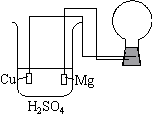## 20.6 Work From a Voltaic Cell

Chemical Concept Demonstrated: Electrochemical work with voltaic or galvanic cells

Demonstration:

 The beaker contains a solution of H2SO4. A copper wire electrode along with a magnesium electrode are inserted into the solution. The wires are connected to a flashbulb. Alternately, a normal light bulb may be used.Observations:

The flashbulb is set off.

Explanation (including important chemical equations):

The standard-state reduction potentials are:

 Mg 2+ (aq) + 2 e- ---> Mg (s) Eo = -2.37 V Cu 2+ (aq) + 2 e- ---> Cu (s) Eo = 0.34 V

The reaction that has an overall positive cell potential is therefore

 Mg (s) ---> Mg 2+ (aq) + 2 e- Eo = 2.37 V 2 H + (aq) + 2 e - ---> H2 (g) Eo =   0.00 V Mg (s) + 2 H + (aq) --->  Mg 2+ (aq) +   H2 (g) Eo cell  = 2.37 V

Electrons flow from the magnesium electrode to the copper electrode through the external circuit, setting off the flashbulb.